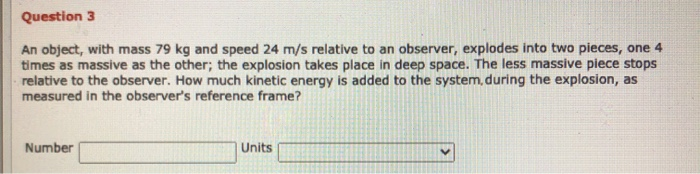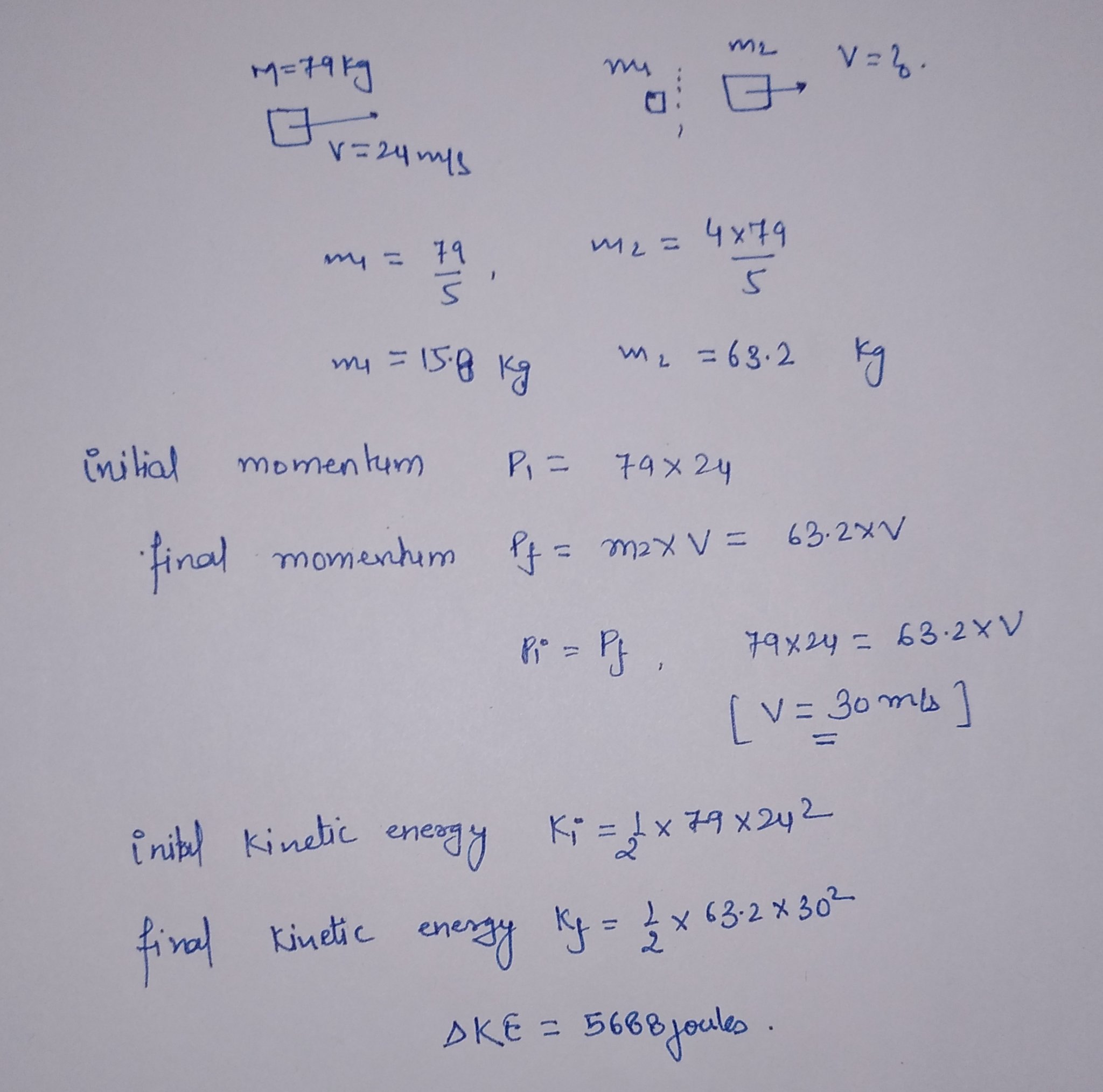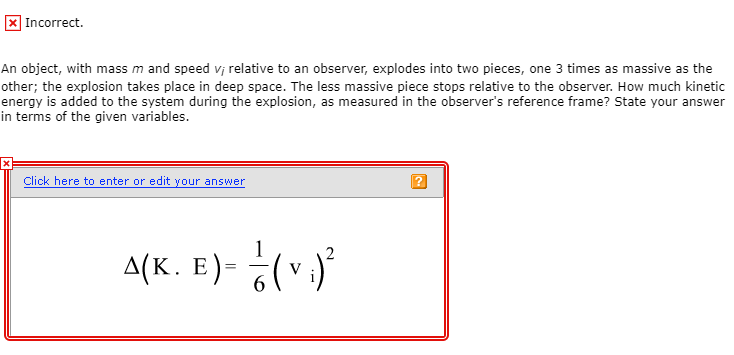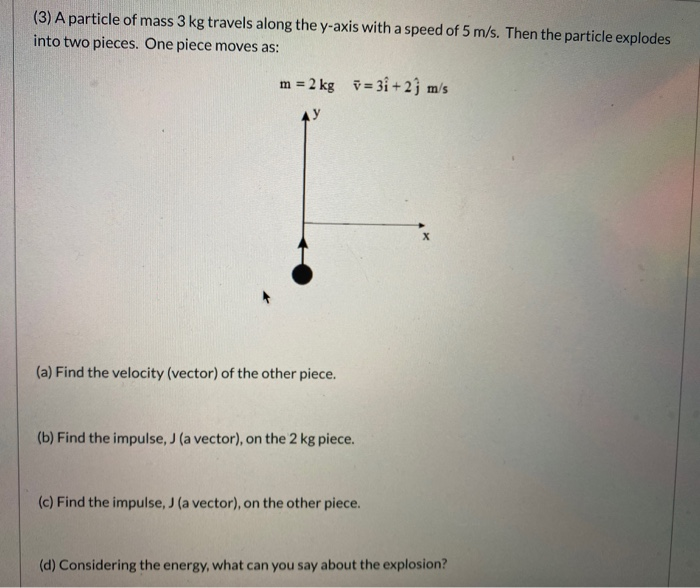Question#### Earn Coins

Coins can be redeemed for fabulous gifts.

Similar Homework Help Questions
• ### An object, with mass 93 kg and speed 23 m/s relative to an observer, explodes into...

An object, with mass 93 kg and speed 23 m/s relative to an observer, explodes into two pieces, one 3 times as massive as the other; the explosion takes place in deep space. The less massive piece stops relative to the observer. How much kinetic energy is added to the system during the explosion, as measured in the observer's reference frame

• ### An object, with mass 67 kg and speed 11 m/s relative to an observer, explodes into...

An object, with mass 67 kg and speed 11 m/s relative to an observer, explodes into two pieces, one 5 times as massive as the other; the explosion takes place in deep space. The less massive piece stops relative to the observer. How much kinetic energy is added to the system during the explosion, as measured in the observer's reference frame?

• ### An object, with mass 42 kg and speed 11 m/s relative to an observer, explodes into...

An object, with mass 42 kg and speed 11 m/s relative to an observer, explodes into two pieces, one 5 times as massive as the other; the explosion takes place in deep space. The less massive piece stops relative to the observer. How much kinetic energy is added to the system during the explosion, as measured in the observer's reference frame?

• ### x Incorrect. An object, with mass m and speed vi relative to an observer, explodes into...x Incorrect. An object, with mass m and speed vi relative to an observer, explodes into two pieces, one 3 times as massive as the other; the explosion takes place in deep space. The less massive piece stops relative to the observer. How much kinetic energy is added to the system during the explosion, as measured in the observer's reference frame? State your answer in terms of the given variables. Click here to enter or edit your answer 4(K. E)-6...

• ### A particle of mass 1.67Ý10-27 kg moves with a speed of 2.6Ý108 m/s relative to an...

A particle of mass 1.67Ý10-27 kg moves with a speed of 2.6Ý108 m/s relative to an observer. What is the kinetic energy of the particle in the rest frame of the observer?

• ### An object with total mass mtotal = 6.4 kg is sitting at rest when it explodes...

An object with total mass mtotal = 6.4 kg is sitting at rest when it explodes into two pieces. The two pieces, after the explosion, have masses of m and 3m. During the explosion, the pieces are given a total energy of E = 45 J. What is the speed of the smaller piece after the collision? What is the speed of the larger piece after the collision? If the explosion lasted for a time t = 0.029 s, what...

• ### (3) A particle of mass 3 kg travels along the y-axis with a speed of 5...(3) A particle of mass 3 kg travels along the y-axis with a speed of 5 m/s. Then the particle explodes into two pieces. One piece moves as: m = 2 kg = 3i+2j m/s (a) Find the velocity (vector) of the other piece. (b) Find the impulse, J (a vector), on the 2 kg piece. (c) Find the impulse, J (a vector), on the other piece. (d) Considering the energy, what can you say about the explosion?

• ### A 121-kg astronaut (including space suit) acquires a speed of 2.30 m/s by pushing off with...

A 121-kg astronaut (including space suit) acquires a speed of 2.30 m/s by pushing off with her legs from a 1800-kg space capsule. Use the reference frame in which the capsule is at rest before the push. Part A What is the velocity of the space capsule after the push in the reference frame? Part B If the push lasts 0.680 s , what is the magnitude of the average force exerted by each on the other? Part C What...

• ### An object of mass 0.9 kg has a speed of 4.2 m/s at position 1 and...

An object of mass 0.9 kg has a speed of 4.2 m/s at position 1 and a kinetic energy of 14.2 J at position 2. Calculate its kinetic energy at position 1, its speed at position 2, and the total work done on the object as it moves from position 1 to position 2. (a) its kinetic energy at position 1 _________J (b) its speed at position 2 __________m/s (c) the total work done on the object as it moves...

• ### Two manned satellites approaching one another, at a relative speed of 0.150 m/s, intending to dock....

Two manned satellites approaching one another, at a relative speed of 0.150 m/s, intending to dock. The first has a mass of 3.50 x 10^3 kg, and the second a mass of 7.50 x 10^3 kg. (a) Calculate the final velocity (after docking) in m/s by using the frame of reference in which the first satellite was orginally at rest. (Assume the second satellite moves in the positive direction. Include the sign of the value in your answer.) (b) What...